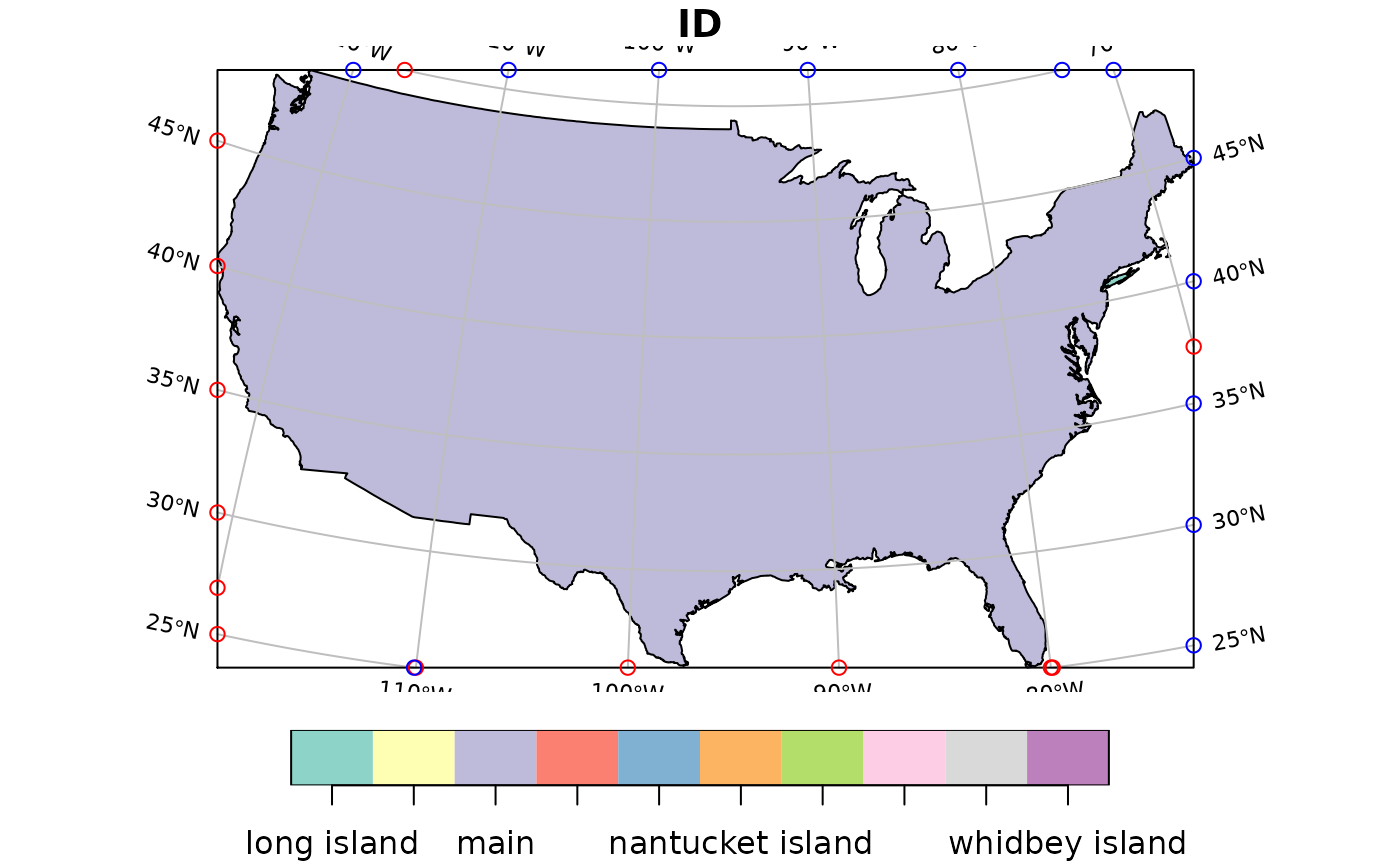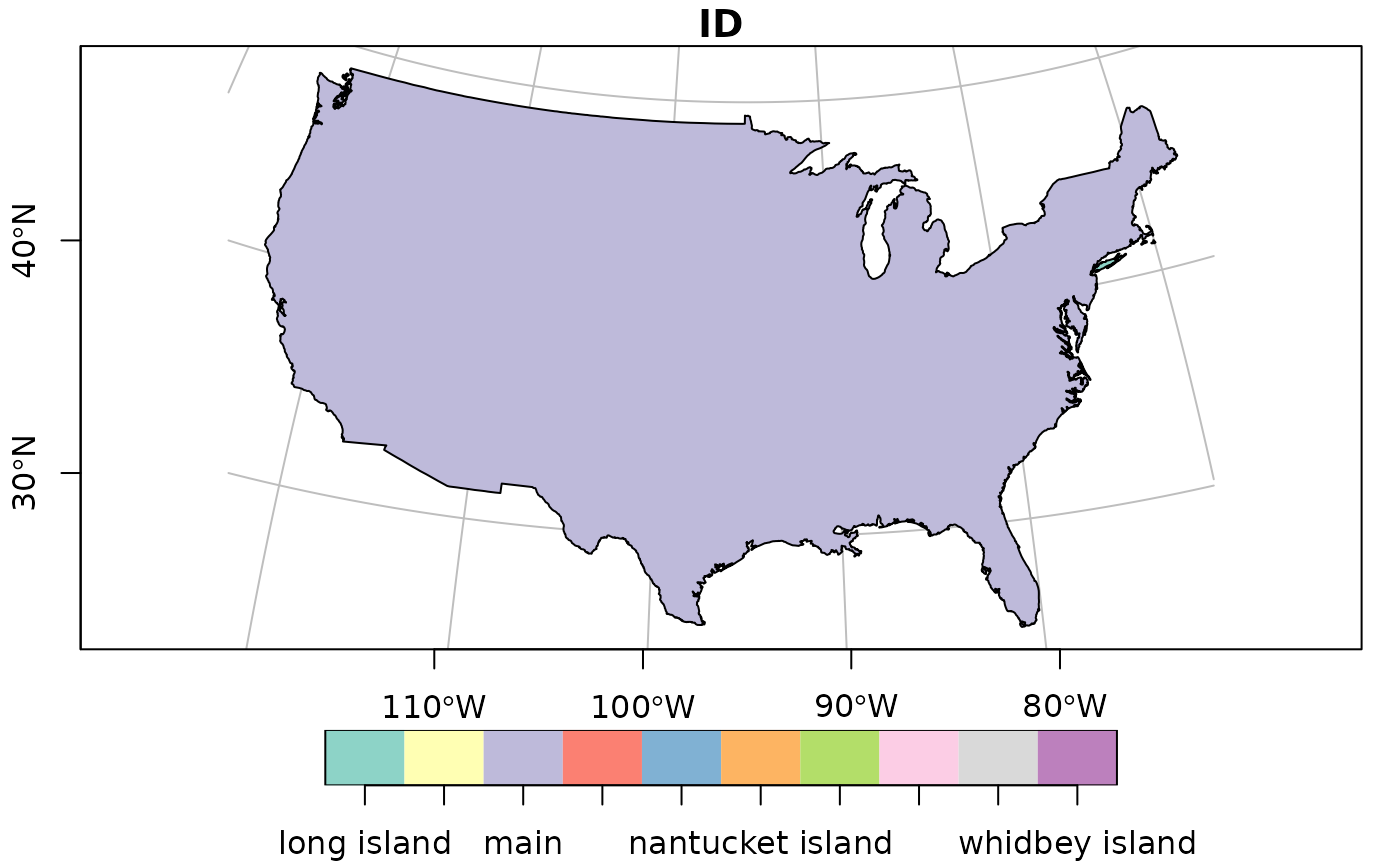Compute graticules and their parameters

## Usage

st_graticule(
x = c(-180, -90, 180, 90),
crs = st_crs(x),
datum = st_crs(4326),
...,
lon = NULL,
lat = NULL,
ndiscr = 100,
margin = 0.001
)

## Arguments

x

object of class sf, sfc or sfg or numeric vector with bounding box given as (minx, miny, maxx, maxy).

crs

object of class crs, with the display coordinate reference system

datum

either an object of class crs with the coordinate reference system for the graticules, or NULL in which case a grid in the coordinate system of x is drawn, or NA, in which case an empty sf object is returned.

...

ignored

lon

numeric; values in degrees East for the meridians, associated with datum

lat

numeric; values in degrees North for the parallels, associated with datum

ndiscr

integer; number of points to discretize a parallel or meridian

margin

numeric; small number to trim a longlat bounding box that touches or crosses +/-180 long or +/-90 latitude.

## Value

an object of class sf with additional attributes describing the type (E: meridian, N: parallel) degree value, label, start and end coordinates and angle; see example.

## Use of graticules

In cartographic visualization, the use of graticules is not advised, unless the graphical output will be used for measurement or navigation, or the direction of North is important for the interpretation of the content, or the content is intended to display distortions and artifacts created by projection. Unnecessary use of graticules only adds visual clutter but little relevant information. Use of coastlines, administrative boundaries or place names permits most viewers of the output to orient themselves better than a graticule.

## Examples

library(sf)
if (require(maps, quietly = TRUE)) {

usa = st_as_sf(map('usa', plot = FALSE, fill = TRUE))
laea = st_crs("+proj=laea +lat_0=30 +lon_0=-95") # Lambert equal area
usa <- st_transform(usa, laea)

bb = st_bbox(usa)
bbox = st_linestring(rbind(c( bb,bb),c( bb,bb),
c( bb,bb),c( bb,bb),c( bb,bb)))

g = st_graticule(usa)
plot(usa, xlim = 1.2 * c(-2450853.4, 2186391.9))
plot(g, add = TRUE, col = 'grey')
points(g$x_start, g$y_start, col = 'red')
points(g$x_end, g$y_end, col = 'blue')

invisible(lapply(seq_len(nrow(g)), function(i) {
if (g$type[i] == "N" && g$x_start[i] - min(g$x_start) < 1000) text(g[i,"x_start"], g[i,"y_start"], labels = parse(text = g[i,"degree_label"]), srt = g$angle_start[i], pos = 2, cex = .7)
if (g$type[i] == "E" && g$y_start[i] - min(g$y_start) < 1000) text(g[i,"x_start"], g[i,"y_start"], labels = parse(text = g[i,"degree_label"]), srt = g$angle_start[i] - 90, pos = 1, cex = .7)
if (g$type[i] == "N" && g$x_end[i] - max(g$x_end) > -1000) text(g[i,"x_end"], g[i,"y_end"], labels = parse(text = g[i,"degree_label"]), srt = g$angle_end[i], pos = 4, cex = .7)
if (g$type[i] == "E" && g$y_end[i] - max(g$y_end) > -1000) text(g[i,"x_end"], g[i,"y_end"], labels = parse(text = g[i,"degree_label"]), srt = g$angle_end[i] - 90, pos = 3, cex = .7)
}))
plot(usa, graticule = st_crs(4326), axes = TRUE, lon = seq(-60,-130,by=-10))
}